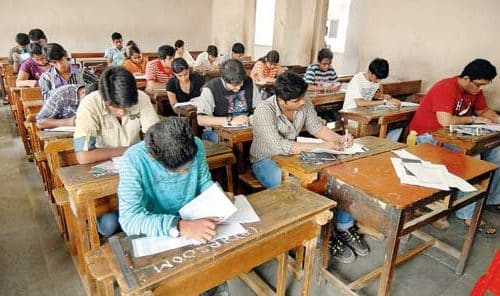• Collection, Classification and Presentation of Statistical Data:
1. Primary and Secondary Data
2. Methods of Data Collection
3. Tabulation of Data
4. Graphs and Charts
5. Frequency Distributions
6. Diagrammatic presentation of Frequency Distributions
• Measures of Central Tendency:
1. Common measures of central tendency, mean, median and mode
2. Partition Values- Quartiles, Deciles, Percentiles
• Measures of Dispersion:
1. Common measures of dispersion, range, quartile deviations, mean deviation and standard deviation
2. Measures of relative dispersion
• Moments, Skewness and Kurtosis:
1. Different types of moments and their relationship
2. Meaning of skewness and kurtosis
3. Different measures of skewness and kurtosis
• Correlation and Regression:
1. Scatter Diagram
2. Simple correlation coefficient
3. Simple regression lines
4. Spearman’s rank correlation
5. Measures of association of attributes
6. Multiple Regression
7. Multiple and Partial Correlation (For three variables only)
• Probability Theory:
1. Meaning of probability
2. Different definitions of probability
3. Conditional probability
4. Compound probability
5. Independent Events
6. Bayes’ Theorem
• Random variable and probability distributions:
1. Random variable
2. Probability functions
3. Expectations and Variance of a random variable
4. Higher moments of a random variable
5. Binomial, Poisson, Normal and Exponential distributions
6. Joint distribution of two random variable (discrete)
• Sampling Theory:
1. Concept of population and sample
2. Parameter and statistic, sampling and non-sampling errors
3. Probability and non-probability sampling techniques (simple random sampling, stratified sampling, multi-stage sampling, multiphase sampling, cluster sampling, systematic sampling, purposive sampling, convenience sampling and quota sampling)
4. Sampling distribution (statement only)
5. Sample size decisions
• Statistical Inference:
1. Point estimation and interval estimation
2. Properties of a good estimator
3. Methods of estimation (Moments method, Maximum Likelihood method, Least Square method)
4. Testing of hypothesis
5. Basic concept of testing
6. Small sample and large sample tests
7. Tests based on Z, t, Chi-square and F statistic, Confidence intervals.
• Analysis of Variance:
1. Analysis of one-way classified data and two-way classified data
2. Time Series analysis- Components of time series, determination of trend component by different methods, measurement of seasonal variation by different methods
• Index Numbers:
1. Meaning of index numbers
2. Problems in the construction of index numbers
3. Types of index number
4. Different Formulae
5. Base shifting and splicing of index numbers
6. Cost of living index numbers
7. Use of index numbers
SHARE
Previous articleSSC-CGL-Tier-2 Exam Syllabus: Paper I & II